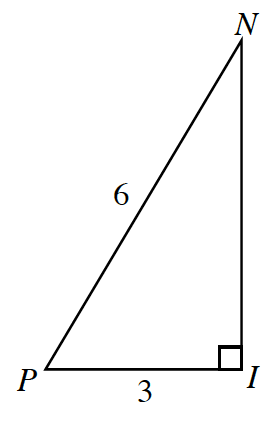### Home > PC > Chapter 3 > Lesson 3.1.2 > Problem3-30

3-30.

Use $\triangle PIN$ to answer the following questions.1. Find the exact length of $\overline{I N}$.

Use the Pythagorean Theorem.

2. Find the ratio for $\cos P$.

$\cos(P) = \frac{\text{adjacent}}{\text{hypotenuse}}$

3. Find the ratio for $\sin P$.

$\sin(P) = \frac{\text{opposite}}{\text{hypotenuse}}$